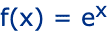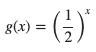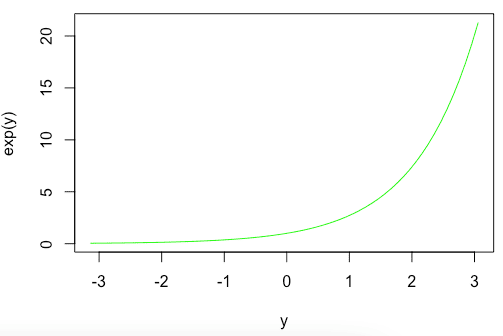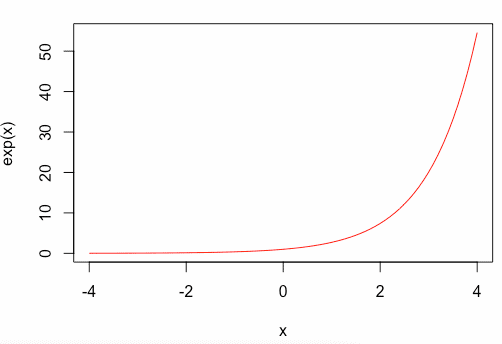# exp function in R: How to Calculate Exponential Value

To create an exponential function, you let the independent variable be the exponent. Exponential functions are solutions to the simplest types of dynamical systems.

The Natural Exponential Function equation is the following.Where e is “Eulers Number” = 2.718281828459

An exponential function can describe growth or decay. For example, the exponential decay function can be described as the following.It gets quickly smaller as x increases.

## exp function in R

The exp() in R is a built-in mathematical function that calculates the exponential value of a number or number vector, e^x. The value of e is approximately equal to 2.71828. The exp() method takes a number as an argument and returns the floating-point number by calculating e^x.

### Syntax

exp(x)

### Parameters

x: It is any valid R number, either positive or negative.

### Return Value

The return value is a floating-point number by calculating e^x.

### Example

Let’s define three numerical values, including floating-point, integer, and double value.

data <- 1
info <- -2
stock <- 1.9

exp(data)
exp(info)
exp(stock)

#### Output

 2.718282
 0.1353353
 6.685894

## Calculate the exponential value of pi in R

The pi is a built-in constant in R. Let’s calculate the exponential value of pi.

exp(pi)

#### Output

 23.14069

Let’s plot the line chart from -pi to +pi.

y <- seq(-pi, pi, by = 0.1)
plot(y, exp(y), typ = "l", col = "green")

#### Output## Calculate the exponential value of a Vector in R

You can define a vector using ( : ) colon operator. To calculate the exponential value of a vector, pass the vector as an argument to the exp() function.

data <- rep(1:5)
exp(data)

#### Output

 2.718282 7.389056 20.085537 54.598150 148.413159

## Plot the exponential value in the range of -4 ~ +4

To plot the exponential value in R, use the plot() function.

y <- seq(-4, 4, by = 0.1)
plot(y, exp(y), typ = "l", col = "green")

#### OutputYou can see that the value is increasing exponentially, and you can clearly see the exponent growth in the line chart.

That is it for exp() function in R language.